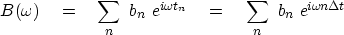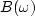Next: FOURIER AND Z-TRANSFORM Up: FOURIER SUMS Previous: Sampled time and Nyquist

## Fourier sum

In the previous section we superposed uniformly spaced frequencies. Now we will superpose delayed impulses. The frequency function of a delayed impulse at time delay t0 is.Adding some pulses yields the Fourier sum'':(19)
The Fourier sum transforms the signal bt to the frequency function.Time will often be denoted by t, even though its units are sample units instead of physical units. Thus we often see bt in equations like (19) instead of bn, resulting in an implied.Next: FOURIER AND Z-TRANSFORM Up: FOURIER SUMS Previous: Sampled time and Nyquist
Stanford Exploration Project
10/21/1998